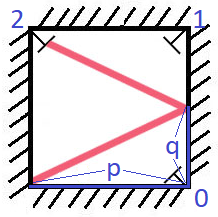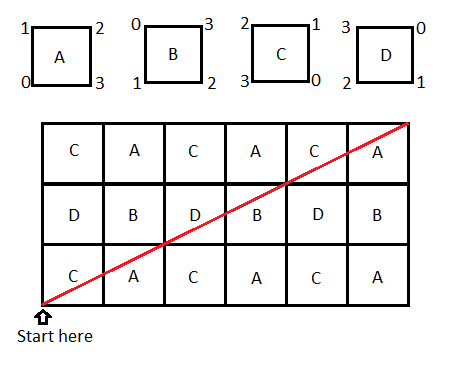# 858 Mirror Reflection

There is a special square room with mirrors on each of the four walls. Except for the southwest corner, there are receptors on each of the remaining corners, numbered `0`, `1`, and `2`.

The square room has walls of length `p` and a laser ray from the southwest corner first meets the east wall at a distance `q` from the `0th` receptor.

Given the two integers `p` and `q`, return the number of the receptor that the ray meets first.

The test cases are guaranteed so that the ray will meet a receptor eventually.

Example 1:``````Input: p = 2, q = 1
Output: 2
Explanation: The ray meets receptor 2 the first time it gets reflected back
to the left wall.
``````

Example 2:

``````Input: p = 3, q = 1
Output: 1
``````

Constraints:

• `1 <= q <= p <= 1000`
 `````` 1 2 3 4 5 6 7 8 9 10 11 12 13 14 15 `````` ``````class Solution: def mirrorReflection(self, p: int, q: int) -> int: if q == 0: return 0 height = q while height < p or height % p: height += q upFlip = height / p rightFlip = height / q topCorner = 1 if upFlip % 2 else 0 return topCorner if rightFlip % 2 else 2````````````class Solution:
def mirrorReflection(self, p, q):
k = 1
while k*q%p:
k += 1
if k%2 == 1 and (k*q//p)%2 == 0:
return 0
if k%2 == 1 and (k*q//p)%2 == 1:
return 1
if k%2 == 0 and (k*q//p)%2 == 1:
return 2
``````